# Using ggplot2 package in R Programming

`ggplot2` also termed as Grammer of Graphics is a free, opensource and easy to use visualization package widely used in R. It is the most powerful visualization package written by Hadley Wickham.
It includes several layers on which it is governed. The layers are as follows: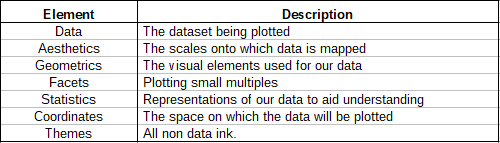#### Building Blocks of layers

Layers with variables of interest are as follows:

• Aesthetics: x axis, y axis, color, fill, size, labels, alpha, shape, line width, line type
• Geometrics: point, line, histogram, bar, boxplot
• Facets: Columns, rows
• Statistics: Binning, smoothing, descriptive, intermediate
• Coordinates: Cartesian, fixed, polar, limits

#### The Dataset

`mtcars`(motor trend car road test) comprises fuel consumption and 10 aspects of automobile design and performance for 32 automobiles and comes pre installed with `dplyr` package in R.

 `# Installing the package ` `install.packages(``"dplyr"``) ` ` `  `# Loading package ` `library(dplyr) ` ` `  `# Summary of dataset in package ` `summary(mtcars) `

#### Performing ggplot2 on dataset

We devise visualisations on `mtcars` dataset which includes 32 car brands and 11 attributes using `ggplot2` layers.

 `# Installing the package ` `install.packages(``"ggplot2"``) ` `  `  `# Loading packages ` `library(ggplot2) ` `library(dplyr) ` `  `  `# Data Layer ` `ggplot(data ``=` `mtcars) ` `  `  `# Aesthetic Layer ` `ggplot(data ``=` `mtcars, aes(x ``=` `hp, y ``=` `mpg, col ``=` `disp)) ` `  `  `# Geometric layer ` `ggplot(data ``=` `mtcars,  ` `       ``aes(x ``=` `hp, y ``=` `mpg, col ``=` `disp)) ``+` `geom_point() ` `  `  `# Adding size ` `ggplot(data ``=` `mtcars,  ` `       ``aes(x ``=` `hp, y ``=` `mpg, size ``=` `disp)) ``+` `geom_point() ` `  `  `# Adding color and shape ` `ggplot(data ``=` `mtcars,  ` `       ``aes(x ``=` `hp, y ``=` `mpg, col ``=` `factor(cyl),  ` `                          ``shape ``=` `factor(am))) ``+` `geom_point() ` `  `  `# Histogram plot ` `ggplot(data ``=` `mtcars, aes(x ``=` `hp)) ``+` `       ``geom_histogram(binwidth ``=` `5``) ` `  `  `# Facet Layer ` `p <``-` `ggplot(data ``=` `mtcars,  ` `            ``aes(x ``=` `hp, y ``=` `mpg,  ` `                ``shape ``=` `factor(cyl))) ``+` `geom_point() ` `  `  `# Separate rows according to transmission type ` `p ``+` `facet_grid(am ~ .) ` `  `  `# Separate columns according to cylinders ` `p ``+` `facet_grid(. ~ cyl) ` `  `  `# Statistics layer ` `ggplot(data ``=` `mtcars, aes(x ``=` `hp, y ``=` `mpg)) ``+`  `                               ``geom_point() ``+`  `       ``stat_smooth(method ``=` `lm, col ``=` `"red"``) ` `  `  `# Coordinates layer: Control plot dimensions ` `ggplot(data ``=` `mtcars, aes(x ``=` `wt, y ``=` `mpg)) ``+` `                               ``geom_point() ``+` `      ``stat_smooth(method ``=` `lm, col ``=` `"red"``) ``+` ` ``scale_y_continuous(``"mpg"``, limits ``=` `c(``2``, ``35``),  ` `                          ``expand ``=` `c(``0``, ``0``)) ``+` `  ``scale_x_continuous(``"wt"``, limits ``=` `c(``0``, ``25``), ` `            ``expand ``=` `c(``0``, ``0``)) ``+` `coord_equal() ` `  `  `# Add coord_cartesian() to proper zoom in ` `ggplot(data ``=` `mtcars, aes(x ``=` `wt, y ``=` `hp, col ``=` `am)) ``+` `                        ``geom_point() ``+` `geom_smooth() ``+` `                        ``coord_cartesian(xlim ``=` `c(``3``, ``6``)) ` `  `  `# Theme layer ` `ggplot(data ``=` `mtcars, aes(x ``=` `hp, y ``=` `mpg)) ``+` `         ``geom_point() ``+` `facet_grid(. ~ cyl) ``+` `        ``theme(plot.background ``=` `element_rect( ` `            ``fill ``=` `"black"``, colour ``=` `"gray"``)) ` `  `  `ggplot(data ``=` `mtcars, aes(x ``=` `hp, y ``=` `mpg)) ``+` `        ``geom_point() ``+` `facet_grid(am ~ cyl) ``+`  `        ``theme_gray() `

Outputs:

• Geometric layer
•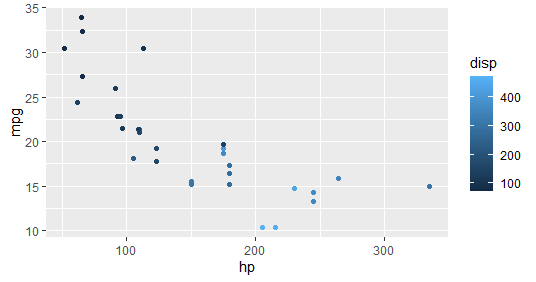• Geometric layer – Adding Size
•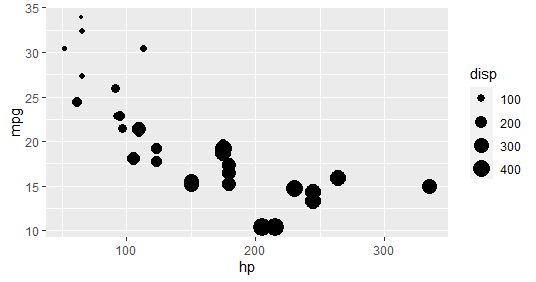• Geometric layer – Adding colour and shape
•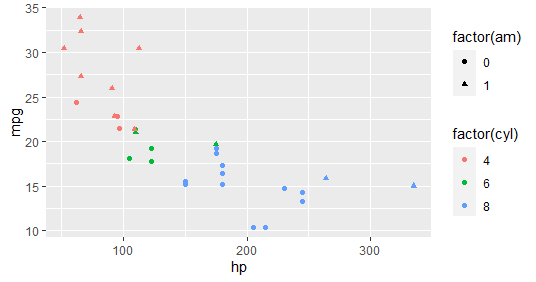• Geometric layer – Histogram plot
•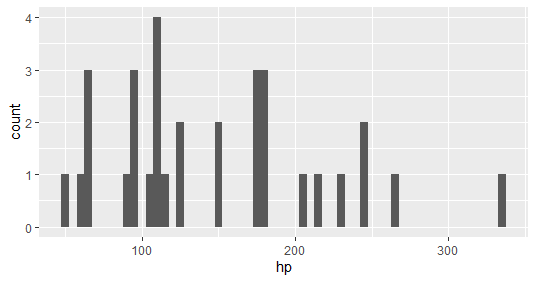• Facet layer – Separate rows according to transmission type
•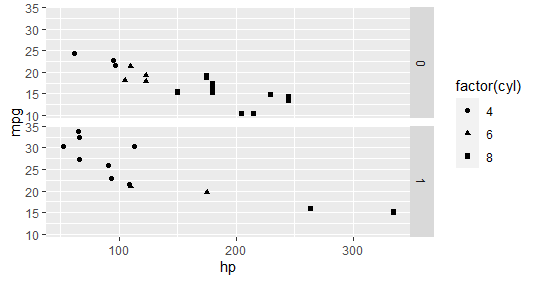• Facet layer – Separate columns according to cylinders
•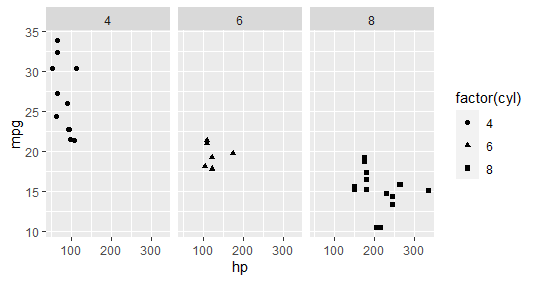• Statistics Layer
•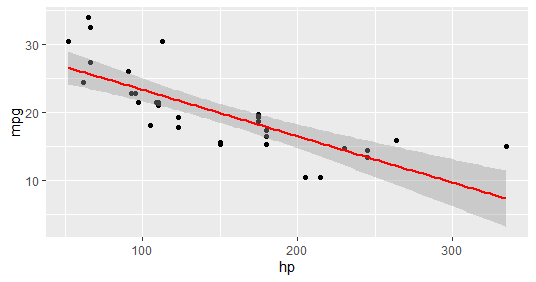• Coordinates layer: Control plot dimensions
•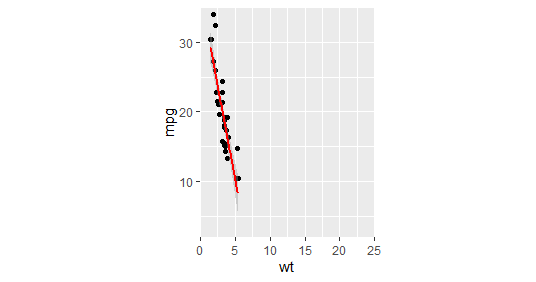• Coord_cartesian() to proper zoom in
•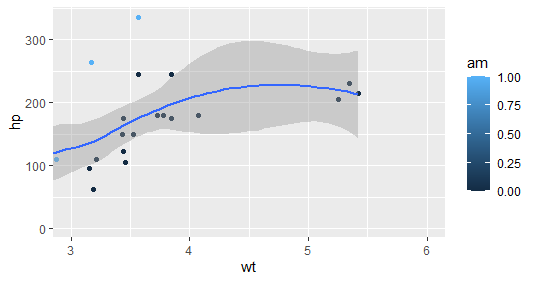• Theme layer – element_rect() function
•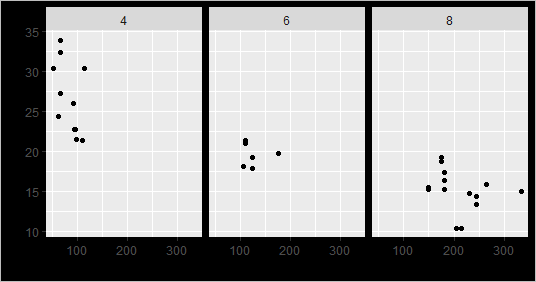• Theme layer
•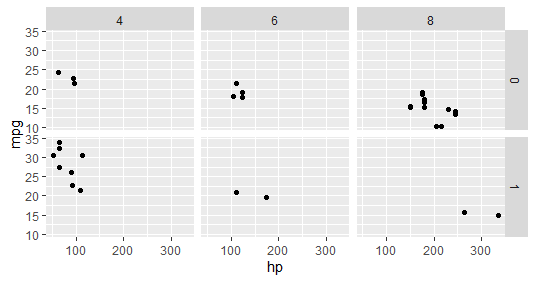`ggplot2` provides various types of visualizations. More parameters can be used included in the package as the package gives greater control over the visualizations of data. Many packages can integrate with the ggplot2 package to make the visualizations interactive and animated.

My Personal Notes arrow_drop_upCheck out this Author's contributed articles.

If you like GeeksforGeeks and would like to contribute, you can also write an article using contribute.geeksforgeeks.org or mail your article to contribute@geeksforgeeks.org. See your article appearing on the GeeksforGeeks main page and help other Geeks.

Please Improve this article if you find anything incorrect by clicking on the "Improve Article" button below.

Article Tags :

Be the First to upvote.

Please write to us at contribute@geeksforgeeks.org to report any issue with the above content.